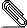# Re: numbering multi-line formulas

```On 04/11/2012 12:12 AM, s nedunuri wrote:
```
Hello, I wonder if anyone can help me with how to number multi-line formulas, as the Help manual appears to be out of date (it refers to menu options that no longer exist). I am running Lyx 2.0.2. I have a multi-line formula that I created via the "Insert Matrix" command. I would like to number each line in the formula. However, when I try this: Edit | Math | there are two choices: Number Whole Formula or Number This Line. However, they seem to be the same thing.
```
```
The problem is that a matrix does not really give you a multi-line formula, it is a one-line formula including a matrix. To get a multiline formula, use one of the aligned environments. I prefer the eqnarray environment. Then you can number each line of the multi-line environment. I will attach a simply LyX file showing first an eqnarray environment, then a matrix environment, both numbered, but the matrix only allows numbering of the whole thing.
```
--

David L. Johnson

Anyone who is capable of getting themselves made President should on
no account be allowed to do the job.numbering.lyx